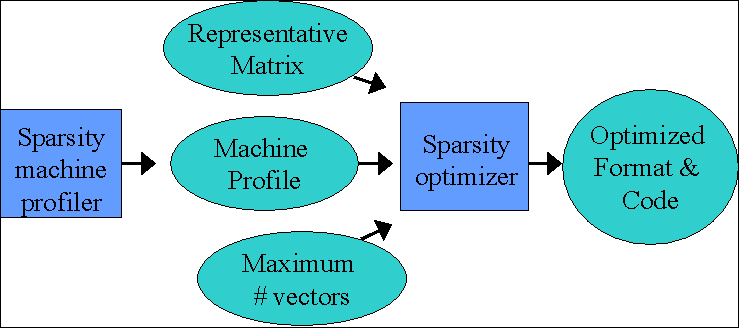Sparsity Eun-Jin Im and Katherine Yelick Sparsity Papers Sparsity Test Matrices

Sparse matrix-vector multiplication is an important computational kernel that arises in scientific simulations, data mining, image and signal processing, and other applications.  It tends to perform poorly on modern processors, because of its high ratio of memory operations to arithmetic operations and the irregular memory access patterns.   Optimizing this algorithm is difficult, because the performance depends on the nonzero structure of the matrix as well as the characteristics of a given memory system.  The Sparsity system is designed to address these problem by allowing users to automatically build sparse matrix kernels that are tuned to their matrices and machines.

Sparsity uses techniques such as register and cache blocking, which are analogous to the idea of blocking (also called tiling) used for dense matrices.  However, the problem is much harder, because the matrix is represented by an indexed structure, so the data structure must be reorganized.  In particular, register blocking is done by filling in zeros of the matrix to create a matrix of equal-sized blocks.  This incurs both storage and computation overhead, but can actually be faster due to reduced indexing overhead and improved locality across two dimensions.

Sparsity automatically generates code for a matrix format the multiplication operation by analyzing the machine and matrix separately, and then combining the results.   The most difficult aspect of optimizing these algorithms is selecting among a large set of possible transformations and choosing parameters, such as block size.  Sparsity generates code for two operations: a sparse matrix times a dense vector and a sparse matrix times a set of dense vectors.  The strategy for choosing register blocks, for example is to use a performance model based on:

1. A matrix-independent, machine-dependent performance profile, plus
2. Matrix-dependent, machine-independent sparsity profile (which may be representative matrix)

The first component is obtained through a search-like process of measuring the performance of a dense matrix in sparse format, blocked for a variety of sizes.  This gives a rough upper bound on the performance that any sparse matrix is likely to exhibit for that block size.  The second measure is used to estimate the number of non-zeros that must be filled into achieve any given level of blocking.  The diagram below gives a rough idea of how information flows in Sparsity.We have also done an extensive performance study of over 40 matrices on a variety of machines. The matrices are taken from various scientific and engineering problems, as well as linear programming and data mining. The machines include the Alpha 21164, UltraSPARC I, MIPS R10000 and PowerPC 604e.  Sparsity is highly effective, producing routines that are up to 3.1 times faster for the
single vector case and 6.2 times faster for multiple vectors, and in a follow-on project, the BeBOP group has found the benefits to be even high on more recent machines like the Pentium IV.

Computer Science Division, University of California at Berkeley
Last updated Sep 2001 Comments to Sparsity Web Maintainer